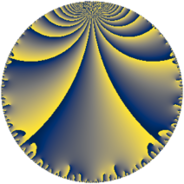# Properties

 Label 50.6.eLevel $50$ Weight $6$ Character orbit 50.e Rep. character $\chi_{50}(9,\cdot)$ Character field $\Q(\zeta_{10})$ Dimension $48$ Newform subspaces $1$ Sturm bound $45$ Trace bound $0$

# Related objects

## Defining parameters

 Level: $$N$$ $$=$$ $$50 = 2 \cdot 5^{2}$$ Weight: $$k$$ $$=$$ $$6$$ Character orbit: $$[\chi]$$ $$=$$ 50.e (of order $$10$$ and degree $$4$$) Character conductor: $$\operatorname{cond}(\chi)$$ $$=$$ $$25$$ Character field: $$\Q(\zeta_{10})$$ Newform subspaces: $$1$$ Sturm bound: $$45$$ Trace bound: $$0$$

## Dimensions

The following table gives the dimensions of various subspaces of $$M_{6}(50, [\chi])$$.

Total New Old
Modular forms 160 48 112
Cusp forms 144 48 96
Eisenstein series 16 0 16

## Trace form

 $$48q + 192q^{4} + 180q^{5} + 72q^{6} + 686q^{9} + O(q^{10})$$ $$48q + 192q^{4} + 180q^{5} + 72q^{6} + 686q^{9} + 80q^{10} + 716q^{11} - 160q^{12} - 784q^{14} - 940q^{15} - 3072q^{16} + 1910q^{17} - 5990q^{19} - 640q^{20} - 4344q^{21} + 4720q^{22} + 12410q^{23} + 4608q^{24} + 23660q^{25} - 15248q^{26} + 27600q^{27} - 3040q^{28} - 17170q^{29} - 14720q^{30} - 18834q^{31} - 56930q^{33} + 11696q^{34} + 28450q^{35} - 10976q^{36} + 3540q^{37} + 13432q^{39} - 1280q^{40} + 37146q^{41} - 43560q^{42} + 1184q^{44} + 75240q^{45} + 28672q^{46} - 17830q^{47} - 2560q^{48} - 54656q^{49} + 8800q^{50} - 85984q^{51} + 22350q^{53} + 16080q^{54} - 64730q^{55} + 12544q^{56} + 18000q^{59} + 78720q^{60} + 67086q^{61} - 159600q^{62} + 132820q^{63} + 49152q^{64} - 195820q^{65} - 52256q^{66} - 90790q^{67} - 212608q^{69} + 180080q^{70} - 122874q^{71} + 121200q^{73} + 281456q^{74} + 672810q^{75} - 102400q^{76} + 434040q^{77} + 170720q^{78} + 113600q^{79} - 28160q^{80} + 115878q^{81} - 582750q^{83} - 174816q^{84} + 107770q^{85} - 26888q^{86} - 911580q^{87} - 56960q^{88} - 357730q^{89} - 282920q^{90} - 216324q^{91} + 451040q^{92} + 79136q^{94} + 135000q^{95} + 18432q^{96} - 123520q^{97} - 206240q^{98} + 235252q^{99} + O(q^{100})$$

## Decomposition of $$S_{6}^{\mathrm{new}}(50, [\chi])$$ into newform subspaces

Label Dim. $$A$$ Field CM Traces $q$-expansion
$$a_2$$ $$a_3$$ $$a_5$$ $$a_7$$
50.6.e.a $$48$$ $$8.019$$ None $$0$$ $$0$$ $$180$$ $$0$$

## Decomposition of $$S_{6}^{\mathrm{old}}(50, [\chi])$$ into lower level spaces

$$S_{6}^{\mathrm{old}}(50, [\chi]) \cong$$ $$S_{6}^{\mathrm{new}}(25, [\chi])$$$$^{\oplus 2}$$